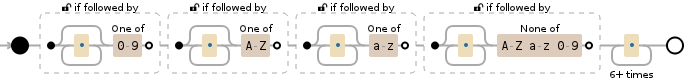# Regex for Password validation in Javascript

Regex Password complexity requires that `any three` of the following four characteristics must be applied when creating or changing a password.

• Alpha characters - at least 1 upper case alpha character
• Alpha characters - at least 1 lower case alpha character
• Numeric characters - at least 1 numeric character
• Special characters - at least 1 special character

I am trying with the following code, but its not working for `special characters`

``````(?=^.{6,}\$)((?=.*\d)(?=.*[A-Z])(?=.*[a-z])|(?=.*\d)(?=.*[^A-Za-z0-9])(?=.*[a-z])|(?=.*[^A-Za-z0-9])(?=.*[A-Z])(?=.*[a-z])|(?=.*\d)(?=.*[A-Z])(?=.*[^A-Za-z0-9]))^.*
``````

I want my regex to be validated against the following 4 cases

Match cases

• P@ssword
• p@ssword1
• p@12345

I think a single regex will be messy in this case. You can easily do something like

``````var count = 0;

count += /[a-z]/.test(password) ? 1 : 0;
count += /[A-Z]/.test(password) ? 1 : 0;
count += /\d/.test(password) ? 1 : 0;
count += /[@]/.test(password) ? 1 : 0;

if(count > 2) {
}
``````
• Can I do it with help of regex, thats the requirement. :( I already achieved it using the above way, but need it in plain Regex. – sivanv Jun 14 '13 at 7:15
• This is regex, but multiple of them. – elclanrs Jun 14 '13 at 7:16
• @sivanv you must also learn, that not always the solution is hammer, even if the problem at the beginning looks like a nail – Danubian Sailor Jun 14 '13 at 7:22
• a single regex here will be too messy and a hell to maintain later – Arun P Johny Jun 14 '13 at 7:24
• I think you could simplify a bit: `count += +/[a-z]/.test(password)` same with the others right? Or simply: `[/[a-z]/,/[A-Z]/,/\d/,/\W/].filter(function(t){ return t.test(pass); }).length > 2` – elclanrs Jun 14 '13 at 7:31

I think that a regex you can use is:

``````(?=^.{6,}\$)(?=.*[0-9])(?=.*[A-Z])(?=.*[a-z])(?=.*[^A-Za-z0-9]).*
``````

I'm not sure why you have so many or operators in your regex but this one matches if:

• `(?=^.{6,}\$)` - String is > 5 chars
• `(?=.*[0-9])` - Contains a digit
• `(?=.*[A-Z])` - Contains an uppercase letter
• `(?=.*[a-z])` - Contains a lowercase letter
• `(?=.*[^A-Za-z0-9])` - A character not being alphanumeric.• But i need any one of the three options, not all the 4. – sivanv Jun 14 '13 at 7:47
• @sivanv Oh, but isn't your regex working? See this. And you can change the password one at a time to check the other ones. – Jerry Jun 14 '13 at 7:55

Use this Regex :

(?=^.{6,10}\$)(?=.\d)(?=.[a-z])(?=.[A-Z])(?=.[!@#\$%^&*()_+}{":;'?/>.<,])(?!.\s).\$**

• not working even the fiddle, could you check again?, even the / is not escaped how would this even work ? – CME64 Jun 14 '13 at 8:59

I think you would need this for all special characters too : [updated to reject space]

``````    \$(document).ready(function(){
\$('input').change(function(){
var count = 0;
var pass = \$(this).val();
count += /[a-z]/.test(pass) ? 1 : 0;
count += /[A-Z]/.test(pass) ? 1 : 0;
count += /\d/.test(pass) ? 1 : 0;
count += /[^\w\d\s]/.test(pass) ? 1 : 0;
(count>2 & !/[\s]+/.test(pass)) ? \$(this).css('background-color','lime'):\$(this).css('background-color','orange');
});

});
``````

and the fiddle : jsFiddle

``````var pattern = new RegExp(/^(?=.*\d)(?=.*[a-z])(?=.*[A-Z])(?=.*[!@#\$%^&*()_+\-=\[\]{};':"\\|,.<>\/?]).{6,}\$/);

if(pattern.test(value)){
return true;
} else {
return false;
}
``````

Its working fine with special character also.